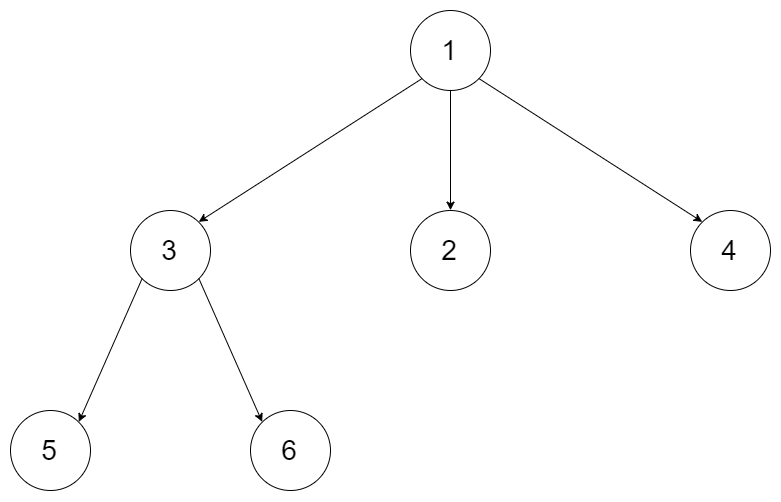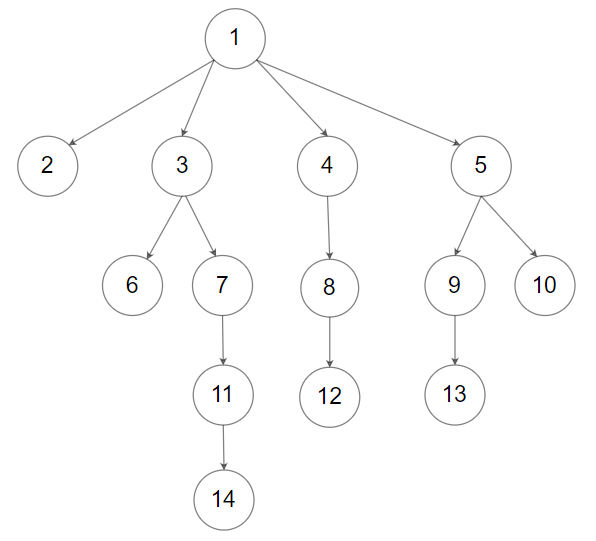### LeetCode• ㊗️
• 大家
• offer
• 多多！

## Problem

Given a n-ary tree, find its maximum depth.

The maximum depth is the number of nodes along the longest path from the root node down to the farthest leaf node.

Nary-Tree input serialization is represented in their level order traversal, each group of children is separated by the null value (See examples).

Example 1:Input: root = [1,null,3,2,4,null,5,6]
Output: 3


Example 2:Input: root = [1,null,2,3,4,5,null,null,6,7,null,8,null,9,10,null,null,11,null,12,null,13,null,null,14]
Output: 5


Constraints:

• The total number of nodes is in the range $[0, 10^4]$.
• The depth of the n-ary tree is less than or equal to 1000.

## Code

/*
// Definition for a Node.
class Node {
public int val;
public List<Node> children;

public Node() {}

public Node(int _val) {
val = _val;
}

public Node(int _val, List<Node> _children) {
val = _val;
children = _children;
}
};
*/

class Solution {
public int maxDepth(Node root) {
if (root == null) return 0;

int maxHeight = 0;
for (Node child : root.children) {
maxHeight = Math.max(maxHeight, maxDepth(child));
}

return 1 + maxHeight;
}
}